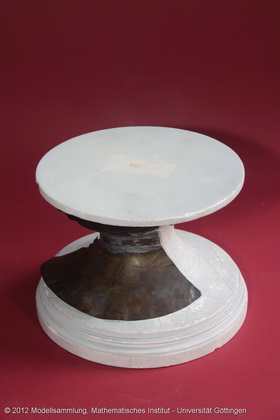Göttingen Collection of Mathematical Models and Instruments

Catenoid

Model 195Size: Constructor: Category: 20 × 0 × 14 Anton von Braunmühl (1853-1908) E V 11-13; E III 5-7; E IV 1-3

Description

Catenoid (rotational surface of the catenary)

This model represents a catenoid, a surface that arises by rotating a curve described by a hanging chain that is only supported by its ends, a so-called catenary. This is the surface that emerges from dipping two parallel, concentric wire circles into a soapy solution. A parametrization is given by the following formula: $x(u,v)=c\cdot \cosh(\frac{v}{c}) \cos(u)$ $y(u,v)= c\cdot \cosh(\frac{v}{c}) \sin(u)$ $z(u,v)=v\ ,$ where $u,v$ are real parameters, and $c\neq 0$ is a real constant.

Jean Baptiste Meusnier showed in the year 1776 that a catenoid is so-called minimal surface, a surface that locally minimizes its area. The catenoid is the only non-planar minimal surface of revolution.

The catenoid is part of a family of parameterized surfaces, it can be converted into the helicoid via continuous deformations.

Catenaries can be found both in nature and architecture, for example in a spiderweb or in the form of the Gateway Arch in St. Louis.

Text written by: Claudia König and Kerstin Bever

Translated by: Lea Renner

Showcase of this model is Case number 39

References

Schilling, Martin(Hrg.): Catalog mathematischer Modelle, Leipzig(Verlag von Martin Schilling) 1911, 7.Auflage, No.243. p. 147.

Separataband M4 im Mathematischen Institut p. 1.

Colding, M.(2011). A Course in Minimal Surfaces.

Eschenburg, J.(2007). Differentialgeometrie und Minimalflächen.